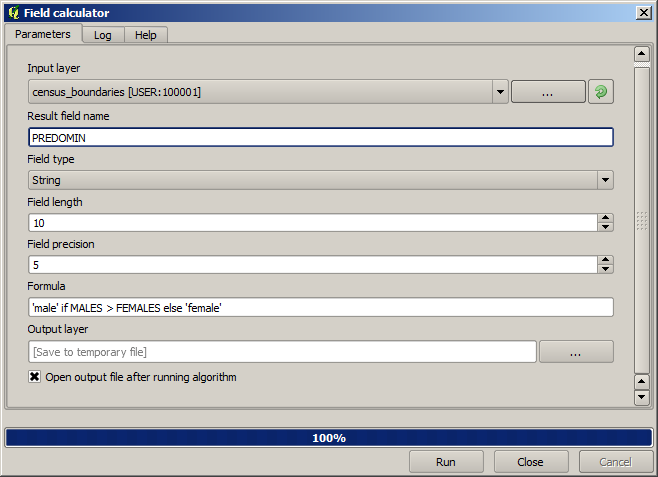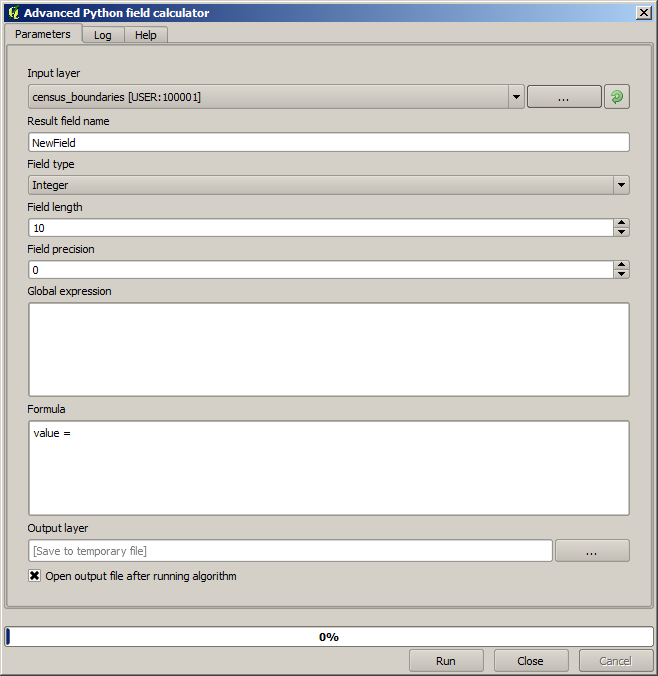Outdated version of the documentation. Find the latest one here.

Nota

We already know how to use the raster calculator to create new raster layers using mathematical expressions. A similar algorithm is available for vector layers, and generates a new layer with the same attributes of the input layer, plus an additional one with the result of the expression entered. The algorithm is called Field calculator and has the following parameters dialog.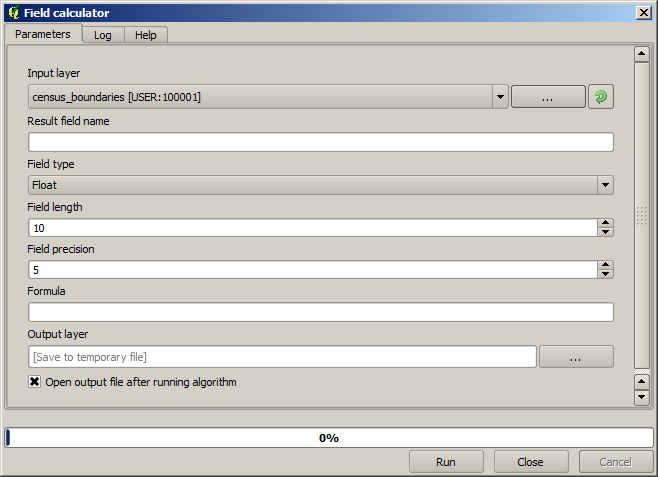Nota

In newer versions of Processing the interface has changed considerably, it’s more powerful and easier to use.

Here are a few examples of using that algorithm.

First, let’s calculate the population density of white people in each polygon, which represents a census. We have two fields in the attributes table that we can use for that, namely WHITE and SHAPE_AREA. We just have to divide them and multiply by one million (to have density per square km), so we can use the following formula in the corresponding field

( "WHITE" / "SHAPE_AREA" ) * 1000000

The parameters dialog should be filled as shown below.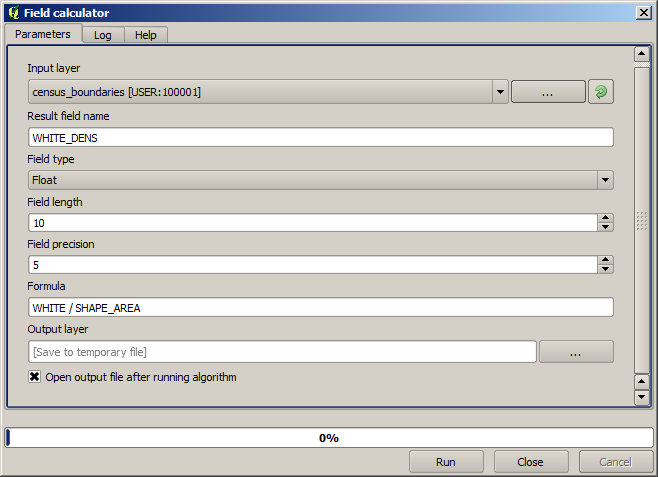This will generate a new field named WHITE_DENS

Agora vamos calcular a proporção entre os campos “MASCULINOS” e “FEMININOS” para criar um novo que indique que a população masculina é numericamente predominante sobre a população feminina.

Entre a fórmula seguinte

"MALES" / "FEMALES"

This time the parameters window should look like this before pressing the OK button.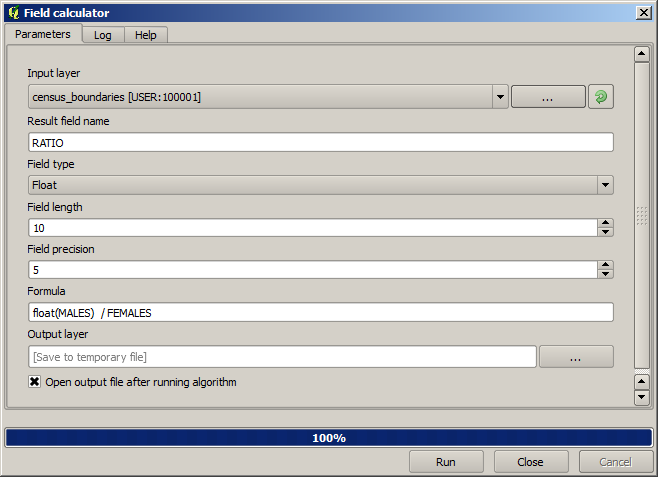In earlier version, since both fields are of type integer, the result would be truncated to an integer. In this case the formula should be: 1.0 *  "MALES"  /  "FEMALES", to indicate that we want floating point number a result.

We can use conditional functions to have a new field with male or female text strings instead of those ratio value, using the following formula:

CASE WHEN  "MALES" > "FEMALES"  THEN 'male' ELSE 'female' END

The parameters window should look like this.